Preferred Citation: Darrigol, Olivier. From c-Numbers to q-Numbers: The Classical Analogy in the History of Quantum Theory. Berkeley:  University of California Press,  1992. http://ark.cdlib.org/ark:/13030/ft4t1nb2gv/Chapter I Concepts of Gas Theory## Chapter I Concepts of Gas Theory

When Planck worked out his radiation theory, he relied on an analogy with Boltzmann's gas theory. The key conceptual issues in the latter theory are best understood in light of their historical source, Maxwell's kinetic theory of gases. The following is a critical discussion of some of Maxwell's and Boltzmann's results.

### Maxwell's Collision Formula

In the mid-nineteenth century James Clerk Maxwell was prominent in developing the kinetic theory of gases, a subject just then beginning to flourish. Like his precursor in the field, Rudolf Clausius, he conceived of a gas as a set of very small "molecules" animated with a continual motion. A molecule in a sufficiently dilute gas was supposed to travel along a straight line, except when it was redirected by short collisions with other molecules or with the walls of a container. Any quantitative theory of the observable effects of such collisions, for instance of pressure or of viscosity, required an evaluation of the number of collisions of a given kind.

In 1866, through a seemingly obvious reasoning, Maxwell gave a precise mathematical expression for this number, later known to German-speaking theorists as the Stosszahlansatz . The corresponding formula turned out to provide the starting point for most subsequent kinetic

 See, e.g., Brush 1976.

8

theories, and these confirmed its validity in many concrete cases. However, the precise formulation of the conditions of its applicability soon became an outstanding conceptual problem of physics. Not only the empirical predictions of the kinetic theory but also, as we shall see, the nature of thermodynamic irreversibility crucially depended on the solution of this problem.

In his "dynamical theory of gases" Maxwell first examined the case of a chemically and spatially homogeneous gas and introduced the following hypothesis of dilution:

We shall suppose that the time during which a molecule is beyond the action of other molecules is so great compared with the time during which it is deflected by that action, that we may neglect both the time and the distance described by the molecules during the encounter, as compared with the time and the distance described while the molecules are free from the disturbing force. We may also neglect cases in which three or more molecules are within each other's spheres of action at the same instant.

In the lack of detailed information on intermolecular forces, Maxwell assimilated the molecules either to hard spheres or to centers of force. In the latter case a collision of two molecules, denoted "1" and "2," may be represented as a simple deflection of "2" in a reference frame fixed to "1" (fig. 1). In the case of central forces the collision "kind" is characterized by the azimuth j of the plane of the trajectory of "2" in this reference frame, and by the angle q between the initial and final relative velocities, which is a definite function of the parameter b (and of the initial relative velocity v2 - v1 ).

Let it be agreed that a collision starts when "2" crosses a conventional plane Z perpendicular to the relative velocity v2 - v1 . Then, for "2" to collide with "1" within the time d t, and with a kind (q , j ), defined with the uncertainty (d q , dj ), it must be located within the "efficient volume" shaded in figure 2. In order to obtain the number of such collisions occurring in a unit volume of a homogeneous gas of identical molecules, Maxwell simply multiplied the measure |v2 - v1 | d t dj b db of this volume by the expression f (v1 )d3v1f (v2 ) d 3v2 , giving the number of pairs of molecules per square unit of volume with velocities v1 and v2 , up to d3v 1 and d3v2 .

 See ibid., 583-640.

 Maxwell 1867, Scientific Papers , 37.

 In his original reasoning Maxwell used the reference frame of the gravity center of the two molecules instead of the reference frame of the molecule "2." The notations used m the sequel are of course not Maxwell's.

9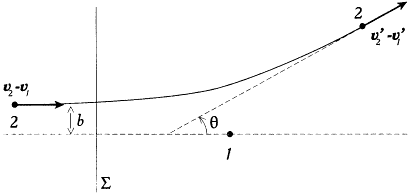Figure 1.
Deflection of a molecule "2" by a molecule "1," as seen from an observer fixed to "1."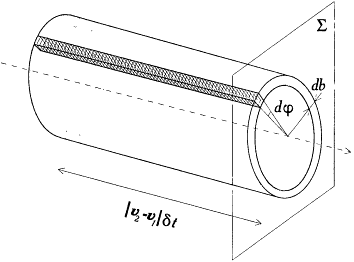Figure 2.
The "efficient volume" for colliding molecules.

This gives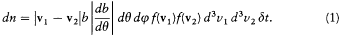In the case of more complex interactions Maxwell used a slightly more general form:10

where dW is an element of solid angle in the direction z defined by q and j , and s has the dimension of a surface.

The seeming naturalness of Maxwell's reasoning obscures an important difficulty. For a given target-molecule "1," the number of molecules in its "efficient volume" must almost always be zero, for it has been implicitly assumed that the time d t is so small that no third molecule perturbs a molecule "2" traveling within this volume. Consequently, the relative spatial distribution of the molecules "2" cannot be considered to be uniform at the scale of the efficient volume. But this distribution depends on the choice of the molecule "1," and an average must be formed with respect to this choice (keeping however the velocity v1 within d3 v1 ). Maxwell's Ansatz implicitly assumes that the distribution resulting from this averaging is uniform, so that the number of pairs of molecules for which the second molecule belongs to the efficient volume of the first is proportional to the value of the efficient volume.

As Boltzmann and Maxwell's British successors would later find, the latter assumption is not always allowed. One can imagine microscopic configurations of the gas for which the number of collisions is not given by Maxwell's formula. For example, let us assume that at a given instant the velocities of nearest neighbor pairs of molecules point toward one another. Then, the number of collisions in a subsequent time interval will greatly exceed the value given by Maxwell, even if the spatial distribution of molecules is as uniform as possible.

This example clearly shows the gap in Maxwell's reasoning: For every choice of the molecule "1" with a velocity within d3 v1 and for a not too small value of d t, there is one molecule "2" in the total (integrated over dW ) efficient volume of "1." Therefore, the average spatial distribution of the molecules "2" (in the sense earlier defined) is far from being uniform; it is more concentrated in the efficient volume than elsewhere.

One would vainly seek for such critical considerations in Maxwell's writings. His Ansatz sounded obvious, and it had the essential advantage of making the number of collisions dependent only on a coarse description of the dynamic state of a gas, namely, a description by a continuous distribution, f (v) or f (r, v) in the configuration space of a molecule. Finer, physically inaccessible details of the molecular description were rendered irrelevant.

This state of affairs allowed Maxwell to draw important consequences from his collision formula. Every transport phenomenon (e.g., transport

 Maxwell 1867, Scientific Papers, 44 .

 Brush 1976, 616-625; Boltzmann 1896b, trans., 40-41.

11

of heat, momentum, etc.) in a gas subjected to external constraints (temperature gradient, pressure gradient, etc.) could be calculated by simply multiplying the elementary transport produced by one collision of a given kind by the number of collisions of this kind, and summing over kind. Most fundamentally, the equilibrium distribution of molecular velocities could be derived from the collision formula. The resulting expression, the so-called Maxwell distribution, had already been obtained by Maxwell in 1860. The new proof of 1866 proceeded in the following way.

Consider two generic elements d3v 1 and d3v2 in the abstract space of molecule velocities, respectively around the velocities v1 and v2 . A sufficient condition of (kinetic) equilibrium is that the number of collisions dn for which the initial velocities belong to these elements, of the kind z , be equal to the number dn ' of collisions for which the final velocities belong to these elements, of the inverse kind z ' (z ' is obtained from z by changing the sign of the angle q between initial and final relative velocities). For these numbers to be finite there must of course be a latitude dW in the definition of z , but we will take it to be negligible in comparison with the latitude in the definition of the direction of v2 - v1 resulting from the finite extension of d3v 1 and d3v2 .

In order to appreciate the consequences of Maxwell's equilibrium condition, one must first note that a precise choice of v1 , v2 , and z implies a definite value of the final velocitiesand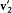, if energy and momentum are conserved during the collision. Indeed, momentum conservation gives, the collision kind gives the orientation of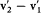with respect to v2 — v1 , and energy and momentum conservation give together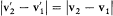; the two last pieces of information give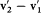, which, combined with the first piece, gives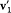and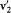. Consequently, direct collisions (contributing to dn ) and reverse collisions (contributing to dn ') are simply related by permuting the roles of the initial and final velocities.

Let us denote byandthe elements in the space of velocities respectively corresponding to the elements d 3v1 and d3v2 for a sharply defined kind of collision z . Then the number dn ' of inverse collisions is given by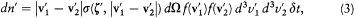a result of Maxwell's Ansatz (2), when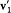and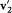are taken as initial velocities, and the selected kind of collision is z '.

 Maxwell 1867, Scientific Papers , 44-46.

12

As shown above,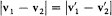, which implies that during a collision the relative velocity u merely rotates. Moreover, the velocity V = (v1 + v2 )/2 of the center of gravity is conserved. Therefore the differential element d3ud3V is conserved. Since d3ud3V = d3v 1d3v2 , the differential element d3v1d 3v2 is also conserved. Finally, the sign of the angle q is clearly irrelevant to the definition of s , which implies s (z ') = s (z ). According to these remarks, the number of inverse collisions can be rewritten asConsequently, the equality of dn and dn ' occurs if and only if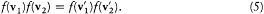Admitting with Maxwell that f cannot depend on the direction of particle velocity, there must exist a function j of v2 such that f (v) = j (v 2 ), and, for any positive numbers x and y , j (x )j (y ) =j (x ')j (y ') if x + y = x' + y '. The latter property is characteristic of exponential functions; hence f must have the formwhich is "Maxwell's distribution" of molecular velocities.

Clearly, this distribution is not modified by collisions inside the gas, as long as these collisions occur at a pace ruled by Maxwell's Ansatz . But can there be other stationary distributions? Maxwell's answer to this question is so brief that it deserves full quotation:

If there were any other [final distribution of velocities] the exchange of velocities represented by OA and OA' [v and v' in the above notation] would not be equal. Suppose that the number of molecules having velocity OA' increases at the expense of OA. Then since the total number of molecules having velocity OA' remains constant, OA' must communicate as many to OA", and so on till they return to OA.

Hence if OA, OA', OA", &c. be a series of velocities, there will be a tendency of each molecule to assume the velocities OA, OA', OA", &c. in order, returning to OA. Now it is impossible to assign a reason why the successive velocities of a molecule should be arranged in this cycle, rather than in the reverse order. If, therefore, the direct exchange between OA and OA' is not equal, the equality cannot be preserved by exchange in a cycle. Hence the direct exchange between OA and OA' is equal, and the distribution we have determined is the only one possible.

 Maxwell 1867, Scientific Papers , 45.

13

Be it wrong, incomplete, or overly condensed, this argument is certainly one of the most impenetrable in all Maxwell's writings. In particular, it is difficult to understand why the balancing of the "direct exchange" between two values of the velocity should be equivalent to the condition dn = dn ', which involves four values of the velocity and pairs of molecules. Even though Maxwell's gas theory rested on sound intuition and skillful mathematics, it failed to provide a convincing proof of the uniqueness of the velocity distribution; and, as we first saw, it left another essential question open, the degree of validity of the collision formula.

### Boltzmann's Irreversible Equations

Boltzmann's first important works were dedicated to an extensive criticism of Maxwell's kinetic theory. In spite of some early doubts, Boltzmann soon adopted the Stosszahlansatz and quickly generalized it to more complex systems. In every application he could verify the validity of results obtained from this hypothesis by an independent method, one based on the general theory of Hamiltonian systems and what was later called the ergodic hypothesis.

When in 1872 Boltzmann questioned Maxwell's laconic reasoning for the uniqueness of the velocity distribution in a dilute gas, he based his alternative proof on the collision formula. The basic idea was simple: knowing the number of collisions of each kind, one could calculate the evolution in time of an arbitrary distribution of velocities and could check whether or not this distribution tended toward Maxwell's. If it did, Maxwell's would then be the unique equilibrium distribution.

### The Boltzmann Equation

With the notation of the preceding section, the number f (v1 ) d3v 1 of molecules in the element d3v 1 of the space of velocities is decreased by all collisions for which one of the initial velocities belongs to d3v 1 ; the other initial velocity and the kind of the collision may be arbitrary. Conversely, this number is increased by all collisions for which one of the final velocities belongs to d3v1 ; here the other final velocity and the kind of the collision may be arbitrary. In mathematical terms this gives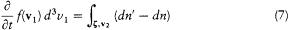See Klein 1973; Brush 1976; Dugas 1959; Darrigol 1988a.

 Boltzmann 1872.

14

or, substituting the expressions (2) and (4) for dn and dn ':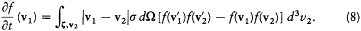Here we need to remember that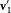, and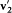are defined by v1 , v2 , and z . This equation is the simplest case of a "Boltzmann equation." The practical importance of this type of equation was considerable, for it permitted Boltzmann to give a systematic derivation of the observable evolution of a thermodynamic system slightly out of equilibrium, including the transport properties already derived by Maxwell by means of a less general method.

### The H-Theorem

However, the most fundamental consequence that Boltzmann drew from his equation was the following theorem on irreversibility: The function of time H defined in terms of the distribution function, f , according tois a strictly decreasing function of time for any choice of f —except Maxwell's distribution, for which H is stationary. This theorem immediately accomplished Boltzmann's initial purpose, a proof of the uniqueness of the equilibrium distribution of velocities. The proof was as follows.

The time derivative of H is given by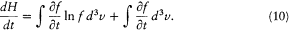The second term of this expression vanishes, since the derivative can be permuted with the integral sign. The substitution of (8) in the first term giveswhere dm is a positive measure in the (v1 , v2 , z )-space, defined as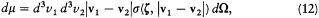15

and where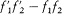stands for. Boltzmann's original proof is considerably simplified by noticing that dm is invariant under a permutation of 1 and 2, and by a permutation of primed and unprimed velocities.

The first of these symmetries gives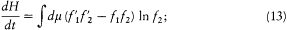the second gives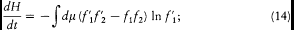and both together giveAdding (11), (13), (14), and (15) and dividing by four gives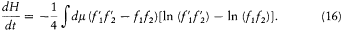The integrand is always positive since the two factors in parentheses always have identical signs (the logarithm being an everywhere increasing function). Therefore dH/dt is always negative, and H is always decreasing. A stable condition occurs only if the integrand vanishes for all values of the variables, that is,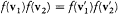for every possible collision. The latter condition is precisely the one leading to Maxwell's distribution. This ends the proof of Boltzmann's H -theorem.

Boltzmann did not fail to notice that -H , calculated for Maxwell's distribution, gave the entropy of a perfect gas (temperatures being measured in energy units). His theorem therefore reproduced the law of entropy increase, insofar as the function -H represented an extension of entropy out of equilibrium. In this way the second principle of thermodynamics could be deduced from kinetic theory, at least for the case of a dilute gas.

### The Nature of Irreversibility

However, Boltzmann soon had to answer an "extremely pertinent" objection raised by his friend and colleague Joseph Loschmidt. Assuming, as

 Boltzmann 1872, BWA 1:345-346.

16

Maxwell and Boltzmann did, the reversibility in time of the dynamics ruling the microscopic evolution of a closed system, one could always imagine microscopic initial conditions leading to a violation of the entropy law. Indeed, to every evolution with increasing entropy there corresponded an evolution with decreasing entropy, obtained by inverting the velocities of all molecules in the final microscopic configuration. It therefore seemed hopeless to try to derive the entropy law from kinetic theory without an ad hoc selection of microscopic initial conditions.

### Entropy and Probability

In the latter conclusion Boltzmann saw nothing but an "interesting sophism." To illustrate his point of view, he considered a gas of hard spheres uniformly spread within the volume of a container (which provides maximum entropy) at the initial time, and he went on to note:

One cannot prove that for every initial choice of the positions and the velocities of the spheres the distribution will be always uniform after a very long time; one can only prove that the number of [microscopic] initial states leading to a homogeneous distribution after a given long time is infinitely greater than the number of initial states leading to a heterogeneous distribution; even in the latter case the distribution would return to homogeneity after a longer time.

In this manner Boltzmann reconciled the second principle with the reversibility of molecular dynamics by arguing that extremely improbable initial conditions could be neglected. He underlined the role of probability considerations in this context: "Loschmidt's argument," he wrote, "shows how intimately the second principle is bound to probability calculus." He further suggested a possible extension: "One might even calculate the probability of various distributions [Zustandverteilung] on the basis of their relative numbers, which might perhaps give rise to an interesting method for the calculation of thermal equilibrium."

In the same year 1877 Boltzmann gave a precise expression to this idea by introducing the quantitative relation between entropy and probability for which he is perhaps most famous. The detailed account of this relation will be postponed to a later section. For the time being it is sufficient

 Boltzmann 1877a, BWA 2: 117; Loschmidt 1876 and subsequent papers. See Brush 1976, 238-240.

 Boltzmann 1877a, BWA 2: 119.

 Ibid., 119; on 121, one reads: "[Loschmidt's] argument shows how intimately the second principle is bound to probability calculus."

 Boltzmann 1877b.

17

to mention a persistent ambiguity in Boltzmann's wording for the exclusion of antithermodynamic processes. In some places these are judged to be "infinitely" rare, and the entropy law is still formulated as being strict. In other places they are said to be "extremely improbable" or "impossible in practice."

### Molecular Chaos

In any case, during these years Boltzmann never explained the exact nature of the probability considerations necessarily entering the proof of this H -theorem. In 1894 he could hear the dissatisfaction of British kinetic theorists concerning this lack of clarity. The discussion led to the concept of "molecular chaos," which had only a transitory importance in Boltzmann's work but a central one in the evolution of Planck's ideas.

For a given partition of the space of molecular velocities into cells, the distribution f (v) can be given a more definite meaning as the list of the numbers Ni representing the number of molecules in the cell i . The corresponding value of H is then defined as the sumIn principle, the molecular dynamics allows one to calculate the evolution in time of these numbers Ni and therefore the exact value of H at every instant, independent of the Boltzmann equation. The corresponding curve H (t ) is extremely chaotic, with a very rapid alternation of increasing and decreasing phases. However, Boltzmann (and, later, more rigorously, Ehrenfest) could show that in a certain sense, which does not need to be specified here, the H -function was more probably decreasing than increasing.

Boltzmann's equation gives the secular evolution of H , that is to say, the average evolution, smearing out local irregularities; it does so thanks to the introduction of a special hypothesis hidden in Maxwell's Ansatz . According to this assumption, called "molecular chaos" by Burbury, certain microscopic "ordered" configurations of the gas must be excluded—for instance, the one already described in the previous section, for which the velocities of closest neighboring molecules point toward each other. Beyond this intuitive but vague characterization, neither Boltzmann nor

 Boltzmann 1877a, BWA 2:120; ibid., 121.

 Burbury 1894 and other British contributions discussed by Brush; Boltzmann 1896b, trans., 40-41. See Brush 1976, 616-625.

 On the H -curve, see Klein 1970c, 114-119.

18

his British friends could provide a general a priori definition of molecular chaos. In the end, they had to content themselves with defining disordered configurations ad hoc , as those for which Maxwell's Ansatz is valid.

### Recurrence

In 1896 Planck's assistant Ernst Zermelo published a new objection to the H -theorem, which left Boltzmann no time to dwell on molecular chaos. Poincaré had proved a few years earlier that any mechanical system confined to a finite region of space would, after a sufficiently long time, return to a configuration arbitrarily near the original one. According to Zermelo, this contradicted not only the H -theorem but also any kinetic theory of heat, since recurrence was never observed in thermodynamics. Boltzmann was particularly irritated by this objection: his previous description of the H -curve did not exclude recurrence; it just made it very improbable. In his opinion, all the objections to the H -theorem could be turned into harmless comments on the statistical validity of the entropy law.

As for Zermelo's contention that recurrence in kinetic theory would contradict the entropy law of thermodynamics, Boltzmann remarked that a typical recurrence time as estimated from kinetic theory was so enormous that "in this length of time, according to the laws of probability, there [would] have been many years in which every inhabitant of a large country committed suicide."

Answering Zermelo's more detailed objections to the shape of the H -curve, Boltzmann improved the description of it by distinguishing eras of entropy increase from eras of entropy decrease in terms of the secular trend of H . For a large number of particles the eras were so long that human observers were confined to a single one, that is, to one direction of time (this direction being defined as that of entropy change).

Coming back to a more realistic time scale, at the end of the century these episodes left no doubt about Boltzmann's understanding of thermodynamic irreversibility: irreversible behavior could be deduced from the kinetic molecular model, but only as a statistical property of the system under consideration. In this way the second principle of thermodynamics lost its absolute character. This had been foreseen by Maxwell in the famous "demon argument" of 1867, and assented to by Gibbs in 1875 in

 Boltzmann 1896b, trans., 40-41.

 Zermelo 1895, 1896; Boltzmann 1896a, 1897a. See Brush 1976, 627-640.

 Boltzmann 1898a, trans., 444.

 Boltzmann 1897a. See Klein 1970c.

19

the following terms: "The impossibility of an uncompensated decrease of entropy seems to be reduced to an improbability." In 1898 Boltzmann quoted this thought at the start of the second part of his Gastheorie .

In the foreword of the same book Boltzmann lamented on the general resistance his ideas met: "I am conscious of being an individual struggling weakly against the stream of the times. But it still remains in my power to contribute in such a way that, when the theory of gases is again revived, not too much will have to be rediscovered." Rather than converting physicists to the kinetic theory, the notion of statistical irreversibility gave new weapons to those, energetists and positivists, who believed that the concepts of energy and entropy were irreducible.

### Summary

Maxwell based his kinetic theory of 1866 on a seemingly obvious derivation of a central quantity: the number of encounters between the molecules of a homogeneous dilute gas. An important property of the resulting expression was that it did not depend on the detailed configuration of the molecules but only on the main quantity of physical interest, the distribution of molecular velocities. In thus eliminating the uncontrollable features of the microscopic description, Maxwell reaped a rich crop: he derived the equilibrium distribution of molecular velocities ("Maxwell's distribution law") and calculated how, through collisions, momentum, kinetic energy, and so on are transferred between contiguous layers of a gas (which accounts for viscosity, thermal conductivity, and other transport phenomena). However, the derivation of the collision formula entailed a hidden assumption of disordered motion, which Maxwell's followers later tried to explain.

Impressed by Maxwell's considerations, Boltzmann greatly extended the generality of the collision formula and the breadth of its applications. Whereas Maxwell had contented himself with the derivation of the final equilibrium distribution (and had given no satisfactory proof of the uniqueness of this distribution), in 1872 Boltzmann managed to derive the time evolution of the distribution of molecular velocities. The corresponding differential equation, which results from Maxwell's collision formula and Conservation laws, involved only the distribution of velocities

 Gibbs 1878, Collected works 1:167; Boltzmann 1896b, trans. 215. For Maxwell's demon, see pp. 22-23 below.

 Boltzmann 1896b, trans., 216.

20

and its time derivative: every uncontrollable feature of the microscopic model was, again, eliminated.

The Boltzmann equation not only simplified access to transport phenomena but also—more fundamentally—implied that the velocity distribution evolves irreversibly toward Maxwell's equilibrium form. Specifically, from the velocity distribution Boltzmann built a certain quantity (later named H by Burbury) which, as a result of the Boltzmann equation, steadily decreases in time until it reaches a minimum value, one that corresponds to Maxwell's distribution. Moreover, the negative of this quantity provided a natural extension of the concept of entropy for a system out of equilibrium; for it increased during irreversible evolution, and, in the equilibrium state, it was identical with the entropy of a perfect gas.

The above result, which constitutes the H -theorem, was repeatedly criticized for conflicting with the general principles of mechanics. In 1876 Loschmidt enunciated his famous paradox: The Boltzmann equation produced irreversible changes, while the equations controlling the underlying molecular dynamics were reversible (symmetric with respect to time reversal). To resolve the conflict, Boltzmann explicitly limited the validity of his equation. He admitted the existence of special molecular configurations for which his equation did not hold; but, he added, such configurations were highly improbable, for they represented only an extremely small fraction of those compatible with given initial macroscopic conditions. In 1877, as a confirmation of this probabilistic view of irreversibility, Boltzmann proposed a direct quantitative relation between entropy and probability, to which I will return later.

In 1894, stimulated by his British colleagues' questions, Boltzmann discussed more precisely the nature of the statistical assumption necessary for the derivation of the Boltzmann equation. This led him to the concept of "molecular chaos" (so named by Burbury). For the Boltzmann equation to hold, excessively "ordered" configurations of the molecules had to be excluded (for instance, those in which every molecule flies toward its nearest neighbor). Aside from such intuitive remarks, Boltzmann contended himself with defining disordered states as states to which Maxwell's collision formula applies. In such a nominalistic guise the concept of molecular chaos could play only a minor role in Boltzmann's writings.

A late attack on the H -theorem came from Planck's assistant Zermelo in 1896. As follows from Poincaré's "recurrence theorem," any finite molecular system has to return to its original macroscopic configuration after a sufficiently long time; therefore, Zermelo concluded, the H -theorem could not be true, even if special "ordered" configurations of the mole-

21

cules were initially excluded. This objection irritated Boltzmann but did not embarrass him: his idea of disorder was meant to be statistical, and average recurrence times were far beyond human observation. But to those who believed in a complete generality of the principles of thermodynamics, Zermelo's argument showed that kinetic theory had to be abandoned, for it was incompatible with strict irreversible behavior.

22Chapter I Concepts of Gas TheoryPreferred Citation: Darrigol, Olivier. From c-Numbers to q-Numbers: The Classical Analogy in the History of Quantum Theory. Berkeley:  University of California Press,  1992. http://ark.cdlib.org/ark:/13030/ft4t1nb2gv/